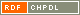# Show document

Title: Quasi m-Cayley circulants Hujdurović, Ademir (Author)http://amc.imfm.si/index.php/amc/article/view/256/196 English Not categorized 1.08 - Published Scientific Conference Contribution IAM - Andrej Marušič Institute A graph ▫$\Gamma$▫ is called a quasi ▫$m$▫-Cayley graph on a group ▫$G$▫ if there exists a vertex ▫$\infty \in V(\Gamma)$▫ and a subgroup ▫$G$▫ of the vertex stabilizer ▫$\text{Aut}(\Gamma)_\infty$▫ of the vertex ▫$\infty$▫ in the full automorphism group ▫$\text{Aut}(\Gamma)$▫ of ▫$\Gamma$▫, such that ▫$G$▫ acts semiregularly on ▫$V(\Gamma) \setminus \{\infty\}$▫ with ▫$m$▫ orbits. If the vertex ▫$\infty$▫ is adjacent to only one orbit of ▫$G$▫ on ▫$V(\Gamma) \setminus \{\infty\}$▫, then ▫$\Gamma$▫ is called a strongly quasi ▫$m$▫-Cayley graph on ▫$G$▫ .In this paper complete classifications of quasi 2-Cayley, quasi 3-Cayley and strongly quasi 4-Cayley connected circulants are given. arc-transitive, circulant, quasi m-Cayley graph 2013 Str. 147-154 1855-3966 519.17 10244273481743 61Document is not linked to any category.

Average score: (0 votes) Voting is allowed only to logged in users.Hover the mouse pointer over a document title to show the abstract or click on the title to get all document metadata.